by Kirby Urner
First posted: October 7, 1997
Last updated: March 25, 2000

The game of quadrays starts with a regular tetrahedron and four rays pointing from its center to the four corners.

We label these rays, or any ray built from these rays, using 4-tuples i.e. four numbers separated by commas and enclosed in parentheses: (a,b,c,d).

If you've used Seymour Papert's kid-friendly Logo computer language, you will recognize the four rays as primitive directions for our turtle. The turtle points in one of these four directions and moves forward by a specific distance.Fig 1: Four basis quadrays to the corners of a regular tetrahedron
 Building new rays to any point in space is accomplished by dragging and dropping copies of already existing rays, starting with these four. You may also "scale" the copies, which means stretch or shrink them using a multiplier (e.g. 3 or 1/2). These multipliers are called "scalars" because they "scale" (resize) the rays (vectors). Always drag and paste a ray with its tail at the tip of some other ray. This is what text books often call vector addition (rays are a type of vector). Thinking of Logo again, we're tracing out the path of a turtle, showing how it got from (0,0,0,0) to some other point in space via some series of moves. One useful format for expressing any quadray as a 4-tuple follows these rules: at least one of these numbers will always be zero, and only positive numbers and zero are needed for any ray, no matter in which direction it points These rules make sense when you realize that: at least one of the four quadrays is always unneeded to reach a certain point (the first rule), and the remaining three will always reach whatever point simply by stretching or shrinking, and adding together tip to tail (the second rule). In other words, only positive scalars are sufficient. Later on in this introduction, we'll look at other ways of "normalizing" (standardizing) quadrays.Fig 2: Tip-to-tail vector addition The rhombus on the left uses two quadrays from the center of the tetrahedron, shown in blue. Notice this rhombus, or parallelogram, has black edges opposite the blue ones. A copy/paste operation applied to either blue ray would give you a black ray. The green ray is the result of blue + a blue clone (i.e. black) -- a new ray from the center of the tetrahedron to this opposite corner of the rhombus. Using 4-tuples we could write: (0,0,0,1) + (0,1,0,0) = (0,1,0,1) So the green vector (or ray, or edge) is (0,1,0,1). This ray shows the most direct route for our turtle, once we have used the primitive directions to define a path to it. Once defined, a ray is available for cloning, just like the primitive ones. Vector addition allows us to quickly define an inventory of useful turtle short cuts for reuse in future vector operations.
 Another way of viewing this same rhombus is as a facet of the rhombic dodecahedron of volume 6. The green edge is part of a cube (volume 3) and the red edge part of an octahedron (volume 4). We know from the concentric hierarchy that: green/red = (cube edge)/(cube diagonal) This will allow us to determine the length of a blue edge using the Pythagorean theorem: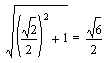Fig 3: Rhombic dodecahedron with inscribe IVM sphere
 Notice that blue + red = other blue in the above figure. Using quadray coordinates we could write: (0,1,0,0) + red = (0,0,0,1) or turtle follows blue, then red, to tip of other blue red = (0,0,0,1) - (0,1,0,0) subtracting (0,1,0,0) from both sides red = (0,-1,0,1) performing vector arithmetic on right side red = (1,0,1,2) simplification to remove negatives from 4-tuple
 In the last step we simplified (0,-1,0,1) by adding an identity of (1,1,1,1). Identities of the form (a, a, a, a) and have no effect when added, but may be used to standardize quadray 4-tuples in accordance with specific rules. In this instance, we're simply adding whatever identity it takes to bring the most negative number up to zero, such that the rest will be positive (or also zero), in conformity with the two rules spelled out above. However, alternative forms of expression may also be used, as discussed below. In the synergetics concentric hierarchy, the rhombic dodecahedron (volume 6) contains the octahedron (volume 4) and cube (volume 3) as sets of long and short face diagonals respectively, as shown at right. These long diagonals also interconnect either the adjacent dense-packed unit-radius spheres of an active vector matrix, or the interspherical voids of an alternate, potentially active vector matrix -- a feature discussed in more detail in my Introduction to Synergetic Crystallography. In light of these facts, the following distance function D(ray) makes a lot of sense: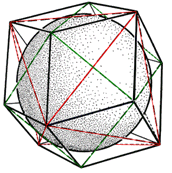Fig 4: Long and short diagonals of the rhombic dodecahedron
 D(1,0,1,2) = 2 two unit radius spheres, connected center-to-center D(0,1,0,1) = root(2) the edge of a cube, given a face diagonal of 2 D(1,0,0,0) = root(6)/2 the center of a regular tetrahedron to any vertex The distance function takes a ray as an argument (in parentheses after the D) and answers with the length of that ray. If we think of rays as objects with a built-in distance method, we might want to think in terms of a computer language that goes something like this: red = object(ray) define red as an object of the predefined ray class red.coords(1,0,1,2) pass coordinates to the method which accepts them d = red.distance( ) assign output of distance method to variable d ? d query for the value of variable d 2 the computer responds Below, we see a different orientation of the quadray tetrahedron, this time inscribed within the volume 3 cube. By following a quadray out to a cube corner, traversing a cube diagonal, and returning to the origin, we define a triangle. For example you might write: (0,0,0,1) + red - (0,0,1,0) = (0,0,0,0)
 Note that in traversing a quadray towards the origin we apply the negative operator, which signifies a change in direction. -(0,0,1,0) is also writable as (0,0,-1,0), bringing the negative inside the parentheses, but then we can apply an additive identity and rewrite this same ray as (1,1,0,1). This is the ray pointing in the opposite direction from (0,0,1,0). Rewriting the above equation we get: (0,0,0,1) + red + (1,1,0,1) = (0,0,0,0)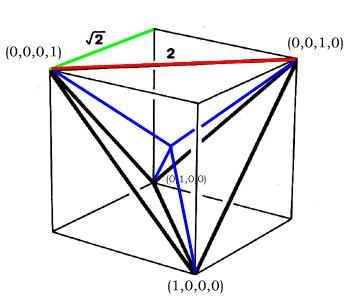Fig 5: Relationship of quadray tetrahedron to volume 3 cube
 (0,0,0,1) + red + (1,1,0,1) = (0,0,0,0) starting equation red = (0,0,0,0) - (0,0,0,1) - (1,1,0,1) subtracting from both sides red = (-1,-1,0,-2) performing vector arithmetic on right side red = (1,1,2,0) simplification to remove negatives from 4-tuple
 Compare the above value of red for the one we had earlier. Both (1,1,2,0) and (1,0,1,2) are 4-tuples with a 0, two 1s and one 2. How many 4-tuples of this form can we write? Here they are: (0,1,1,2) (0,1,2,1) (0,2,1,1) (1,0,1,2) (1,0,2,1) (1,1,0,2) (1,1,2,0) (1,2,0,1) (1,2,1,0) (2,0,1,1) (2,1,0,1) (2,1,1,0) Note that if we take any of the above 4-tuples, put a negative in front, and simplify, we get another 4-tuple from the same set. For example -(1,1,2,0) = (-1,-1,-2,0) = (1,1,0,2) and -(2,1,1,0) = (-2,-1,-1,0) = (0,1,1,2). What this shows is that the above set of 12 rays defines all six edges of the volume 1 tetrahedron going in both directions. Looking at these 12 rays pointing from (0,0,0,0), we see they point to the corners of a vector equilibrium or cuboctahedron of volume 20. All 24 circumferential edges of this shape are likewise clones of the central radial vectors, showing that all edges of the IVM (isotropic vector matrix) are traversed by a turtle programmed to move forward and back in the 12 directions defined by the edges of the regular tetrahedron.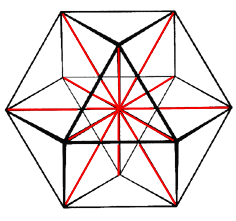Fig 6: 12 radials to the corners of a cuboctahedron
 Using a similar reasoning process, we can see that all possible pairs of quadrays added together give green vectors, of which there are six: (1,1,0,0) (0,1,1,0) (0,0,1,1) (1,0,0,1) (1,0,1,0) (0,1,0,1)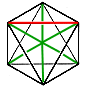Fig 7: Octahedron And once again bidirectionality is included, in that the negative of any of the above is also contained within the original set. For example: -(1,0,0,1) = (-1,0,0,-1) = (0,1,1,0). Collected at the origin (0,0,0,0), these 6 rays point from the center of a regular octahedron (volume 4) to its 6 vertices. The rays themselves all have a length of root(2). A turtle constrained to move only along these rays would define an XYZ type of vector matrix (or lattice) of volume 3 cubes. This fact that the six permutations of {1,1,0,0} gives us six rays in a mutually orthogonal XYZ orientation suggests a method for converting XYZ 3-tuples in and out of quadray 4-tuples. Lets refer to the four quadrays as v-rays, because they point to the tetrahedron's vertices, and to the six vector sums (or linear combinations) of v-rays as e-rays, because they point through the tetrahedron's six edges.
 The figure at right shows v-rays and e-rays together. Note that an e-ray, (0,1,0,1) for example, reaches the mid-edge of the tetrahedron half way to its final distance at root(2) from the origin. In other words, given the labeling scheme at right, the following equalities hold true: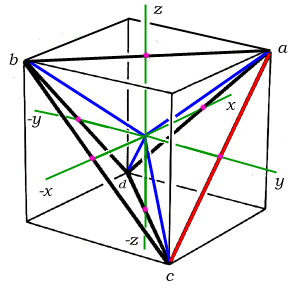Fig 8: Quadray tetrahedron mid-edges as cube-face centers Given the above identities, we can substitute for x, y and z into the standard XYZ distance function to obtain an equivalent function in terms of a,b,c and d. This gives the D(ray) function specified above where, again, we expect the following values:
 D(1,0,1,2) = 2 two unit radius spheres, connected center-to-center D(0,1,0,1) = root(2) the edge of a cube, given a face diagonal of 2 D(1,0,0,0) = root(6)/2 the center of a regular tetrahedron to any vertex The function in question is: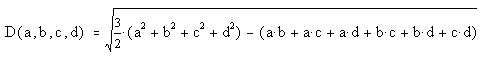The above distance function operates on quadrays regardless of how they're nomalized. However, sometimes if we get more specific about the form of normalization, we can simplify our quadray expressions considerably. Let's examine how this works in this case of a distance formula. Because any (k,k,k,k) denotes four quadrays pointing equally in the four basic directions, it serves as an "additive identity" i.e. (k,k,k,k) may be added to any quadray without changing its direction or magnitude. Given this freedom to add any (k,k,k,k) to any quadray without changing it, we have the option to normalize quadrays according to a variety of rules. For example, we can require that a+b+c+d=0 for any quadray (a,b,c,d), and get there by adding some (k,k,k,k). Simply set the value of k to -(a+b+c+d)/4 in this case. Tom Ace came up with the above method for "normalizing to zero" in the process of deriving an alternative, and much less complicated expression for the length of a quadray. His expression for distance, using this "zero-based" format, is simply: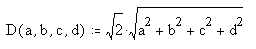where the root(2) multiplier in front scales the result in accordance with the stipulation that our "home base" tetrahedron should have edges = 2 (and consequently D(1,0,0,0) = root(6)/2). Note that we might want to express our vector lengths using IVM sphere diameters as units, or the basis quadrays themselves. In other words, if our quadray tetrahedron is defined to have unit edges, such that IVM sphere radii are valued at 1/2, then we will use different constants in our distance and quadray-to-XYZ conversion formulae. Likewise, we might choose to define D(1,0,0,0)=1, in which case the tetrahedron's edges will need to be multiplied accordingly. To prevent confusion, it may help to think in terms of units like feet and inches. The exact form of our expressions depends on whether we want to use R, D or Q (quadray) as our units of measure. In this introductory essay, I use R units. Elsewhere, I have used D units. Other authors have used Q units. These alternative zero- or one-based normalizations may well feature a negative operator, as in -(1,0,0,0). Geometrically speaking, the negatives of the four basis quadrays correspond to the quadrays of the "inverse tetrahedron", which point outward from (0,0,0,0) at 180 degrees to those of the "home base" or "all positive" tetrahedron. As demonstrated above, negative numbers are perfectly meaningful within quadray 4-tuples, but we always have the option to renormalize such that they go away, if we so desire.
 We can also look at quadrays as edges of the A module of volume 1/24. At right is a one-quarter tetrahedron divided into six A mods (left and right handed). The apex of this quarter tet is also the center of the full-sized unit volume tetrahedron, and so the three edges connecting this apex to the corners of the base are quadrays. A second triangle extended off one side of the quarter tet completes a parallelogram, providing the same rhombic face (with long and short diagonals) as used above.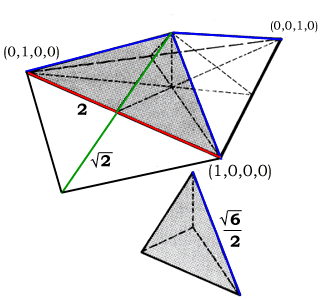Fig 10: Quadrays in relationship to the A-module For further reading: Graphics developed from original artwork in Synergetics by R. Buckminster Fuller in collaboration with E.J. Applewhite Synergetics on the Web maintained by Kirby Urner